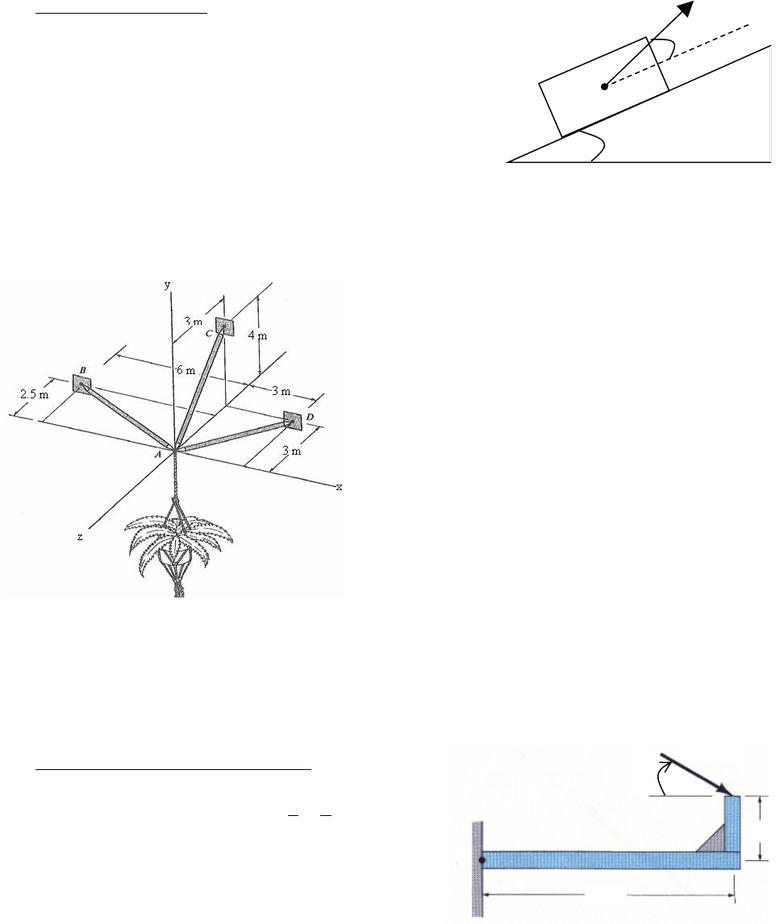# ENGG 202 Lecture Notes - Lecture 1: Cross Product, Unit Vector, Contact Force

20 views4 pagesENGG 202 Tutorial 5 February 5/6 2013
All problems are from old exams
30o
20o
P
A
Review – Equilibrium
1) The block has a weight of 100 N which acts at the
centre A. The surface is frictionless so the contact force is
normal to the inclined surface. What is the magnitude of
the force P required to hold the block in the equilibrium
position shown? ANS: 53.2 N
Solution Strategy: draw the FBD of the block.
There are 3 forces acting on the FBD, the weight (vertical), normal force perp to the surface, and
force P. Easiest would be to use a rotated set of axis (parallel and perp to slope)
Find the components of all the forces, write the eqns of equil and solve.
2) Determine the force acting along each strut (AB,
AC, and AD) necessary to hold the 200 N plant pot in
equilibrium. Points B and D lie in the x-z plane. Point
C lies in the y-z plane.
ANS: FAC = 250 N (away from A), FBA = 115 N
(toward A), FDA = 150 N (toward A)
Solution Strategy: draw the FBD of point A. it has 1
known (the weight) and 3 unknown forces (AD, AC,
AB) acting on it. The directions of the unknown forces
can be assumed as you wish (toward the point or away
from the point). Find the components of the forces
(magnitude is unknown so simply multiply the unit
vectors for each by a variable representing the force
magnitude.)
Sum the forces in the x, y, and z directions equal to zero and solve for the 3 unknown force
magnitudes. Negative answers tell you if your assumed directions are wrong (and therefore
opposite to the correct direction).
Moment about a point using scalars
3) Determine the direction θ (0 < θ < 180o) of the
40 N force F so that F produces (a) the maximum
moment about point A and (b) the minimum
ANS: 63.4o, 153o
Solution Strategy: The maximum moment will occur when F is perpendicular to the line
connecting A to the point of application of F. The minimum moment will occur when the line of
action of F passes through point A.
F
2 m
4 m
A
θ
Unlock document

This preview shows page 1 of the document.
Unlock all 4 pages and 3 million more documents.